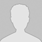#学习小专题

## 离子方程式的四步配平法

1）要判断添加的缺项一般是反应介质。如酸（H+）、碱（OH）或水（H2O）等，需要根据反应的具体情况判断添加。

2）H+和OH不可能添加在同一边，因H+和OH不可能大量共存。

3）若反应物中添加H+或OH离子，则生成物中往往需添加水；若反应物中添加水，则生成物中往往需添加H+或OH离子。

4）添加H+或OH离子时，不必考虑与反应物能否共存，但一定要考虑与生成物能否共存，不能共存，就不能添加。

[例1] 配平离子方程式：Fe2++ClO――Fe3++Fe(OH)3+Cl

2Fe2++1ClO――Fe3++Fe(OH)3+1Cl

2Fe2++1ClO+H2O――Fe3++Fe(OH)3+1Cl

2Fe2++1ClO+H2O――4/3Fe3++2/3Fe(OH)3+1Cl

6Fe2++3ClO+3H2O==4Fe3++2Fe(OH)3↓+3Cl

[例2] 配平离子方程式：MnO4+Fe3O4――Fe3++Mn2+

1MnO4+5Fe3O4+  ――15Fe3++1Mn2++

1MnO4+5Fe3O4+      ――15Fe3++1Mn2++H2O

MnO4+5Fe3O4+48 H+==15Fe3++Mn2++24H2O

1.MnO4+ H2O2+=Mn2++ O2+  H2O

2.PbO2+  Mn2++  H+=  Pb2++  MnO4+

3.MnO4+  H2S+    =Mn2++  S+  H2O

4.Cr2O72+  I    =  Cr3++  I2+

5.An +    BY=   Bm+   AX

6.Fe3++  Mn2++  H2O=  MnO2+  Fe2++

7.CrO42+  S2+   =  Cr(OH)3+  S2O32+

8.Cr2O72+   H2O2+    =    Cr3++  O+  H2O

9.BiO3+  Mn2++     =  MnO4+   Bi3++

10. SX2+    BrO3+    OH=    SO42+    Br+    H2O

1.2MnO4+ 5H2O2+6 H+=2Mn2++5 O2+ 8H2O

2.5PbO2+ 2Mn2++4H+=5Pb2++2MnO4+ 2 H2O

3.2MnO4+ 5H2S+6 H+=2Mn2++ 5S+8H2O

4.Cr2O72+6I+14H+= 2Cr3++ 3 I2+7 H2O

5.myAn +xmnBY= xnBm+ mnyAX

62Fe3++  Mn2++  2H2O=  MnO2+2Fe2++4 H+

7.8CrO42+ 6S2+23 H2O =  8Cr(OH)3+ 3S2O32+22 OH

8.Cr2O72+3H2O2+8 H+=2Cr3++3O+7H2O

9.5BiO3+2Mn2++14 H+= 2MnO4+5Bi3++7H2O

10. 3SX2+（3x+1）BrO3+ 6（x-1）OH= 3xSO42+（3x+1）Br+3（x-1）H2O

(责任编辑：)

------分隔线----------------------------

------分隔线----------------------------

 离子方程式配平中缺项的填写 离子方程式的四步配平法文档 互换反应离子方程式配平 氧化还原离子方程式配平及训练 离子方程式配平中缺项的填写 缺项离子方程式配平• 全部评论（0
还没有评论，快来抢沙发吧！